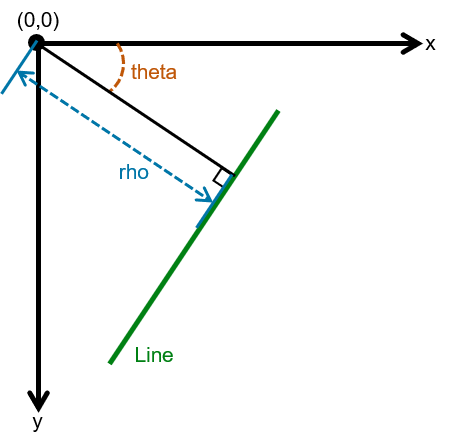# hough

Hough 变换

## 语法

[H,theta,rho] = hough(BW)
[H,theta,rho] = hough(BW,Name,Value)

## 说明

[H,theta,rho] = hough(BW) 计算二值图像 BW 的标准 Hough 变换 (SHT)。hough 函数旨在检测线条。该函数使用线条的参数化表示：rho = x*cos(theta) + y*sin(theta)。该函数返回 rho（沿垂直于线条的向量从原点到线条的距离）和 theta（x 轴与该向量之间的角度，以度为单位）。该函数还返回 SHT H，它是一个参数空间矩阵，其行和列分别对应于 rho 和 theta 值。有关详细信息，请参阅算法。

[H,theta,rho] = hough(BW,Name,Value) 使用名称-值参数计算二值图像 BW 的 SHT 以影响计算。

## 示例

RGB = imread('gantrycrane.png'); I = im2gray(RGB);

BW = edge(I,'canny');

[H,T,R] = hough(BW,'RhoResolution',0.5,'Theta',-90:0.5:89);

subplot(2,1,1); imshow(RGB); title('gantrycrane.png'); subplot(2,1,2); imshow(imadjust(rescale(H)),'XData',T,'YData',R,... 'InitialMagnification','fit'); title('Hough transform of gantrycrane.png'); xlabel('\theta'), ylabel('\rho'); axis on, axis normal, hold on; colormap(gca,hot);RGB = imread('gantrycrane.png'); I = im2gray(RGB);

BW = edge(I,'canny');

[H,T,R] = hough(BW,'Theta',44:0.5:46);

figure imshow(imadjust(rescale(H)),'XData',T,'YData',R,... 'InitialMagnification','fit'); title('Limited Theta Range Hough Transform of Gantrycrane Image'); xlabel('\theta') ylabel('\rho'); axis on, axis normal; colormap(gca,hot)## 输入参数

### 名称-值参数

Hough 变换 bin 沿 rho 轴的间距，指定为介于 0 和 norm(size(BW)) 之间（不包含两者）的正数。

SHT 的 Theta 值，指定为数值向量，其中包含在 [-90, 90) 范围内的元素。

## 输出参数

Hough 变换矩阵，以大小为 nrho×ntheta 的数值矩阵形式返回。行和列对应于 rhotheta 值。有关详细信息，请参阅算法

x 轴和 rho 向量之间的角度，以度为单位，以数值矩阵形式返回。有关详细信息，请参阅算法

## 算法

rho = x*cos(theta) + y*sin(theta)SHT 是参数空间矩阵，其行和列分别对应于 rhotheta 值。SHT 中的元素表示累加器元胞。最初，每个元胞中的值为零。然后，对于图像中的每个非背景点，为每个 theta 计算 rhorho 舍入到 SHT 中最近的允许行。该累加器元胞递增。在此过程结束时，SHT(r,c) 中的 Q 值表示 xy 平面中的 Q 个点位于 theta(c)rho(r) 指定的线条上。SHT 中的峰值表示输入图像中可能存在的线条。

Hough 变换矩阵 H 大小为 nrho×ntheta，其中：

nrho = 2*(ceil(D/RhoResolution)) + 1，且
D = sqrt((numRowsInBW - 1)^2 + (numColsInBW - 1)^2)
rho 的值在 -diagonaldiagonal 范围内，其中
diagonal = RhoResolution*ceil(D/RhoResolution)

ntheta = length(theta)